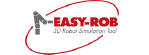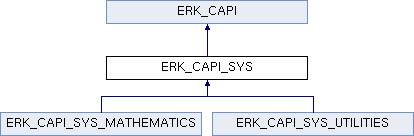EASY-ROB™ Kernel  v8.603
ERK_CAPI_SYS Class Reference

Method class for mathematical calculations, simulation status, units. More...

`#include <erk_capi.h>`

Inheritance diagram for ERK_CAPI_SYS:## Static Public Attributes

static ERK_CAPI_SYS_UTILITIES erk_capi_sys_utilities
Method class for helping functions, color conversion, etc. More...

static ERK_CAPI_SYS_MATHEMATICS erk_capi_sys_mathematics
Method class for mathematical calculations, multiplications of homogeneous matrices, conversion Euler angle, triangle calculations, formula parser, etc. More...Static Public Attributes inherited from ERK_CAPI
Method class to administrate this Robotics Simulation Kernel. More...

static ERK_CAPI_DEVICES erk_capi_devices
Method class to create, attach, update devices, for kinematics calculations and for motion planning and -execution. More...

static ERK_CAPI_SIM erk_capi_sim
Method class for simulation settings. More...

static ERK_CAPI_AUTOPATH erk_capi_autopath
Method class for collision free path planning. More...

static ERK_CAPI_TARGETS erk_capi_targets
Method class for paths and tags. More...

static ERK_CAPI_GEO erk_capi_geo
Method class to handle 3D Geometry Data. More...

static ERK_CAPI_SYS erk_capi_sys
Method class for mathematical calculations, simulation status, units. More...

## Detailed Description

Method class for mathematical calculations, simulation status, units.

## ◆ erk_capi_sys_mathematics

 ERK_CAPI_SYS_MATHEMATICS ERK_CAPI_SYS::erk_capi_sys_mathematics
static

Method class for mathematical calculations, multiplications of homogeneous matrices, conversion Euler angle, triangle calculations, formula parser, etc.

## ◆ erk_capi_sys_utilities

 ERK_CAPI_SYS_UTILITIES ERK_CAPI_SYS::erk_capi_sys_utilities
static

Method class for helping functions, color conversion, etc.

The documentation for this class was generated from the following file: